Function Repository Resource:

# Cyclide

Compute expressions for Dupin cyclide surfaces

Contributed by: Wolfram Staff (original content by Alfred Gray)
 ResourceFunction["Cyclide"][a,c,k,{u,v}] computes the elliptic–hyperbolic cyclide with parameters a,c and k, parameterized in terms of the variables u and v. ResourceFunction["Cyclide"][a,k,{u,v}] computes the parabolic cyclide with parameters a and k, parameterized in terms of the variables u and v. ResourceFunction["Cyclide"][a,…,k] represents an operator form that can be applied to variables u and v.

## Details and Options

A Dupin cyclide or cyclide of Dupin is any geometric inversion of a standard torus, cylinder or double cone. Charles Dupin discussed his discovery of cyclides in his 1803 dissertation.
Dupin cyclides are surfaces that are envelopes of spheres. The spheres are then tangent to the cyclides along circles, which are the curvature lines.
The parametrization of elliptic–hyperbolic cyclides is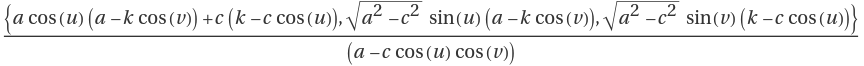, where a is the semimajor axis, c is the linear eccentricity of the ellipse and k is the radius of the generating sphere at the co-vertices of the ellipse (0,±b), where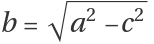.
The parametrization of parabolic cyclides is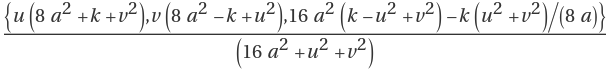, where a determines the shape of the associated parabolas and k determines the ratio of the diameters of the two holes.
Elliptic–hyperbolic cyclides are a parametrization of Dupin cyclides of radius k whose focal sets are the ellipse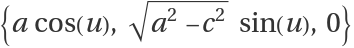and the hyperbola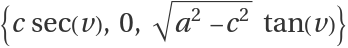.
Parabolic cyclides are a parametrization of Dupin cyclides of radius k whose focal sets are the parabolas {u,0,-u2/(8 a)+a+k } and {0,v,v2/(8 a)-a-k}.
Dupin cyclides have also been investigated by A. Cayley and J. C. Maxwell.
Dupin cyclides have applications in computer-aided design (CAD).

## Examples

### Basic Examples (6)

Get the equation for a ring elliptic‐hyperbolic cyclide:

 In:=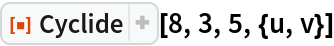Out=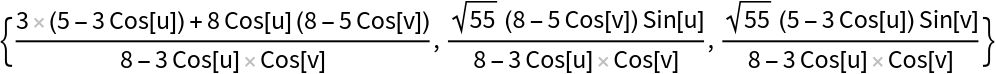Plot it:

 In:=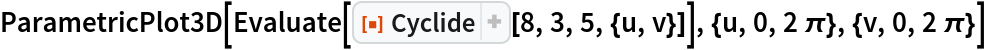Out=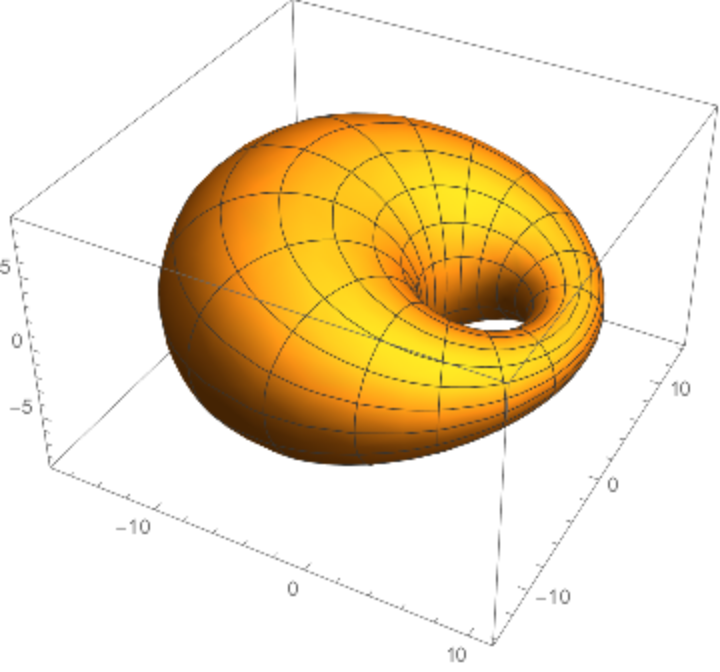A horn cyclide:

 In:=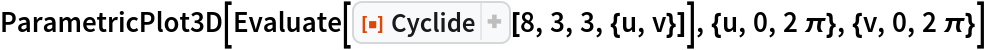Out=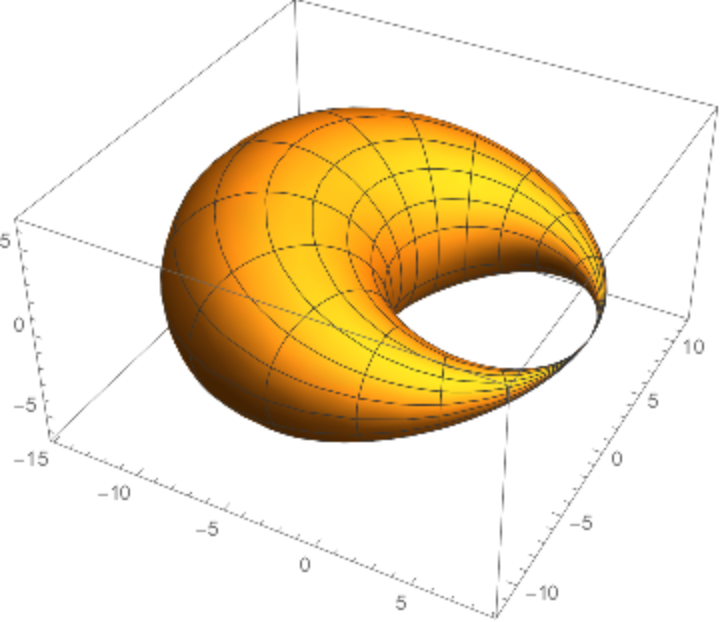Another horn cyclide:

 In:=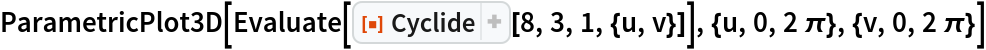Out=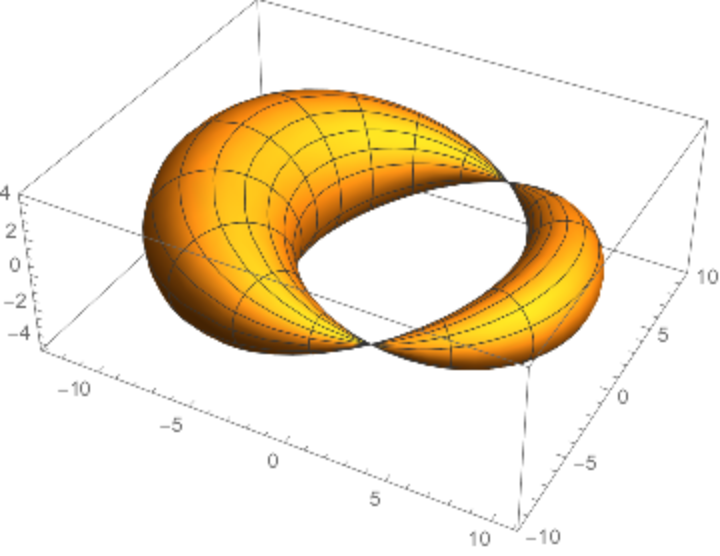A spindle cyclide:

 In:=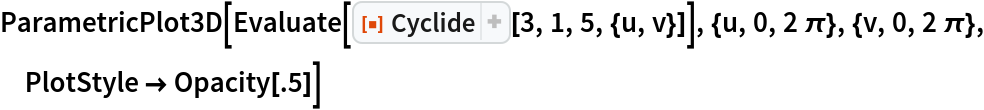Out=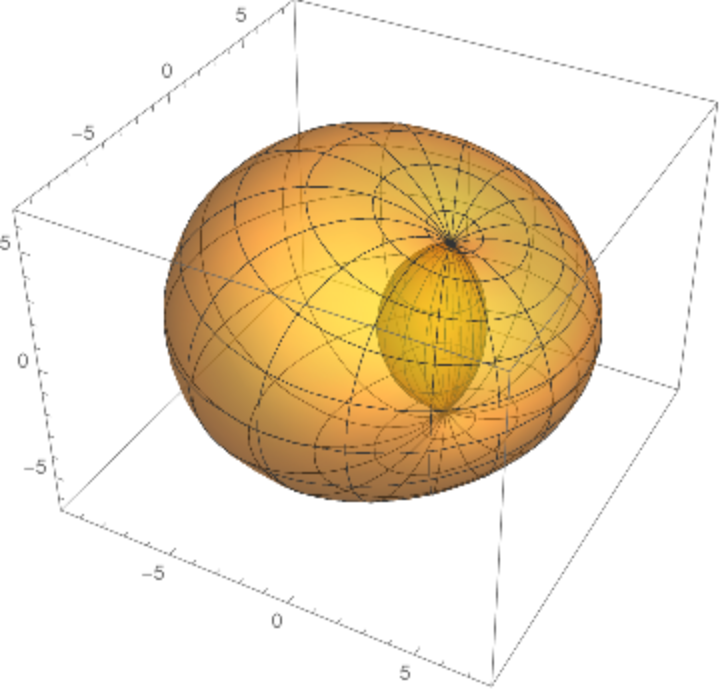A ring cyclide with no hole:

 In:=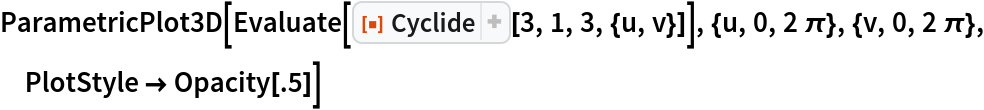Out=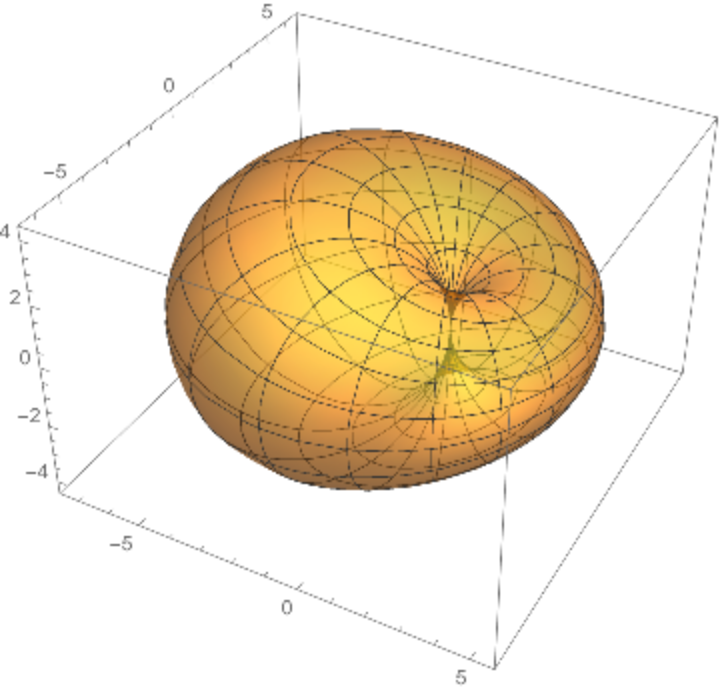A ring parabolic cyclide:

 In:=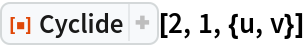Out=In:=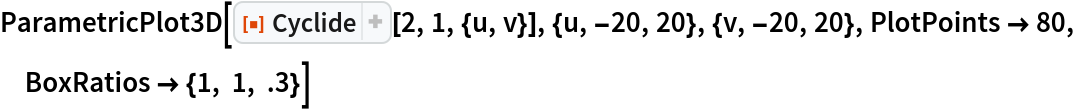Out=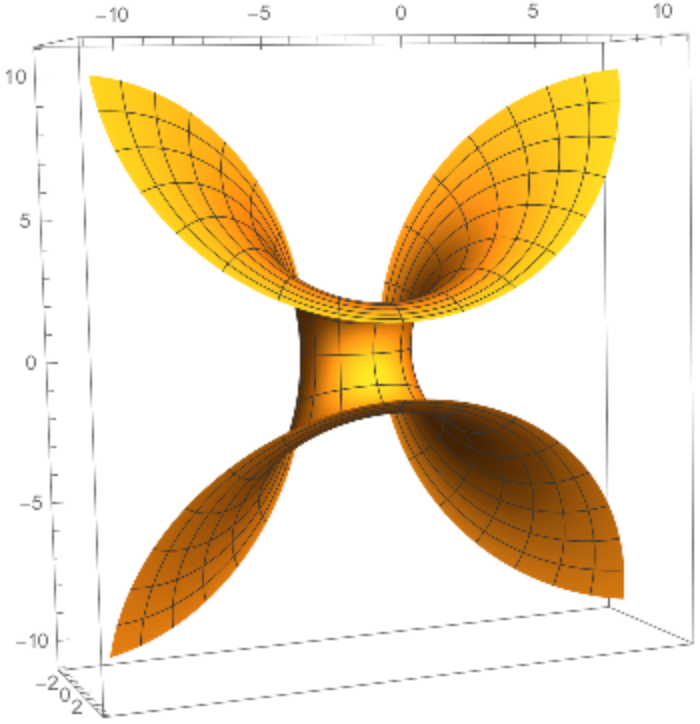The implicit representation of the cyclide:

 In:=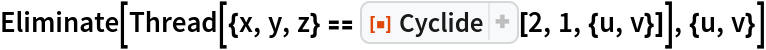Out=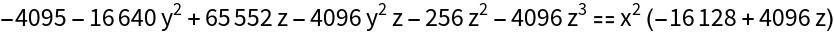Plot the implicit equation and rotate to see the holes:

 In:=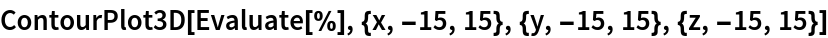Out=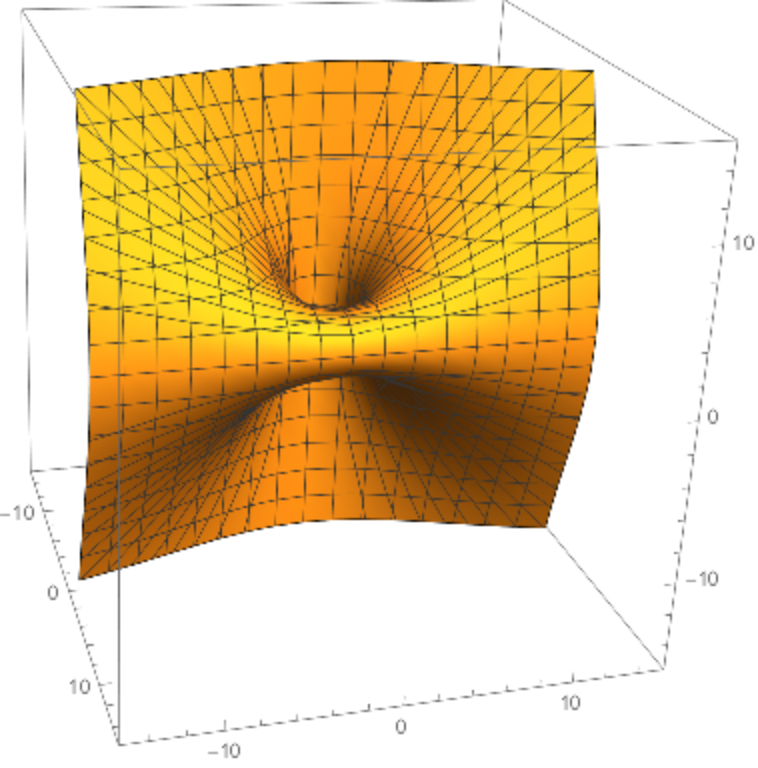A horn parabolic cyclide:

 In:=Out=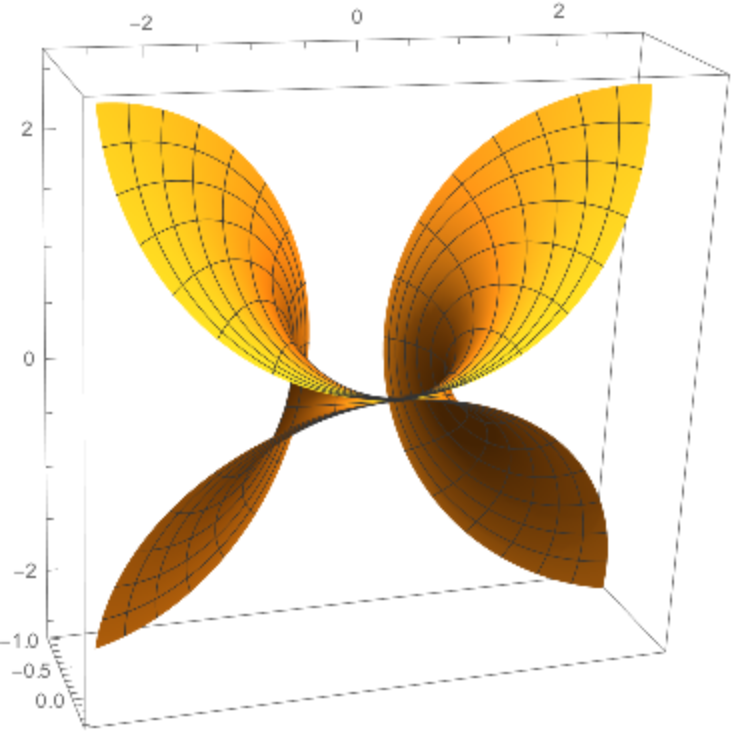Another horn parabolic cyclide:

 In:=Out=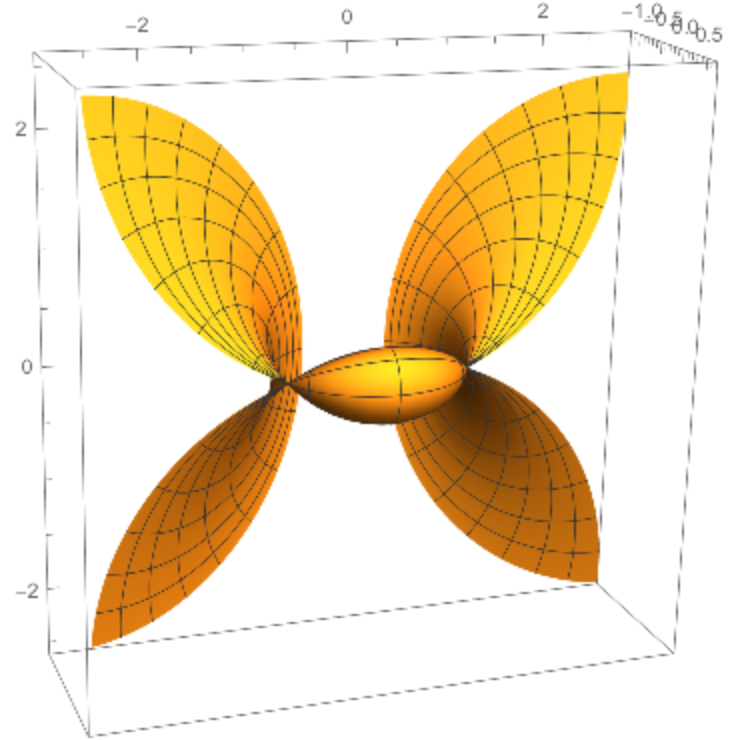### Properties and Relations (4)

Compute the focal set for elliptic–hyperbolic cyclides:

 In:=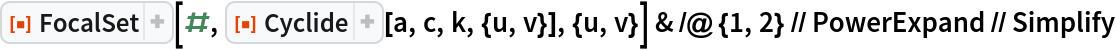Out=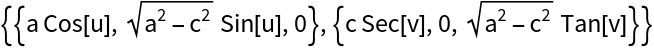Compute the focal set for the parabolic case:

 In:=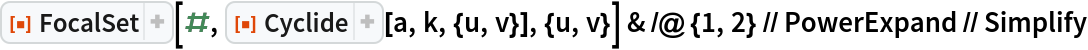Out=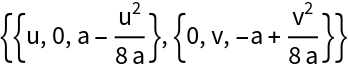Code for generating a cyclide together with its focal curves:

 In:=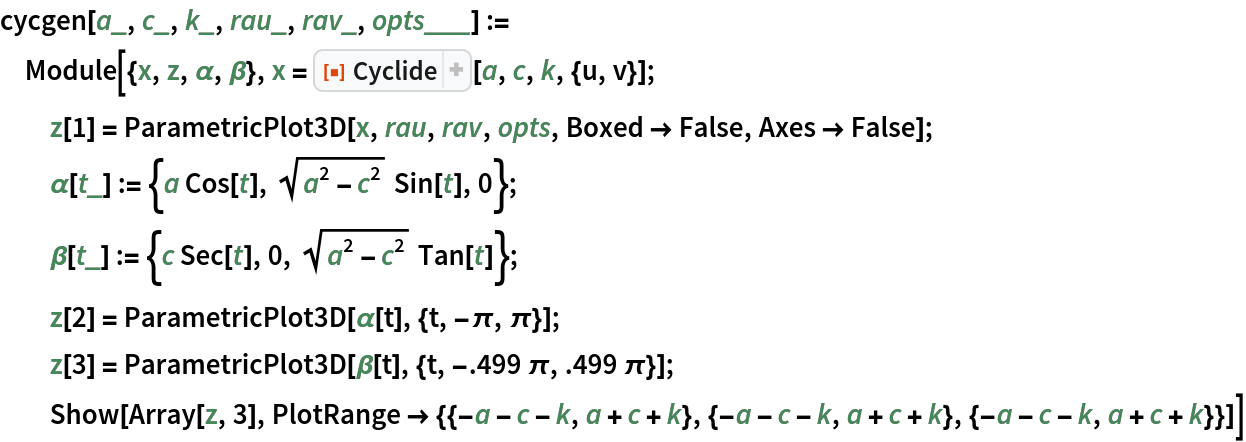The ring cyclide with its focal curves:

 In:=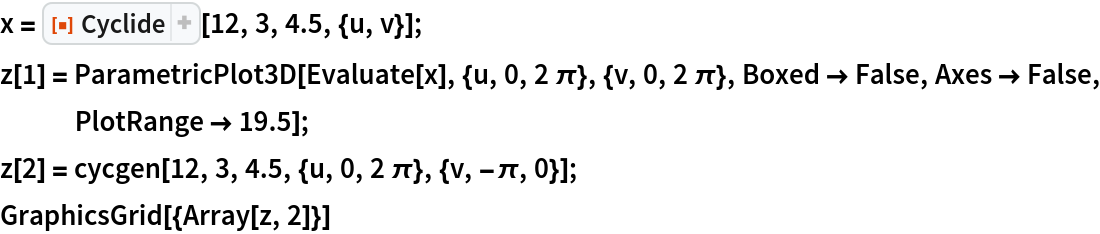Out=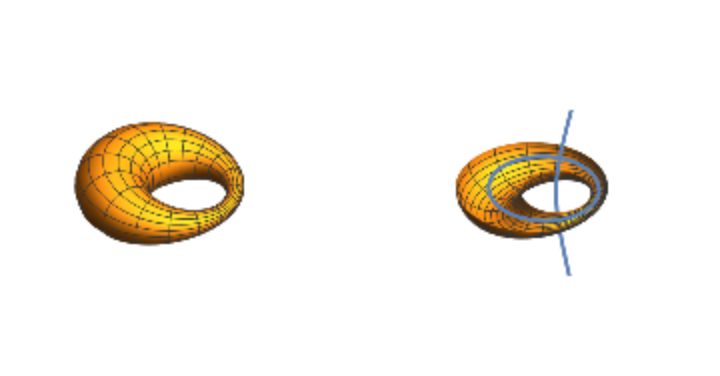The horn cyclide with its focal curves:

 In:=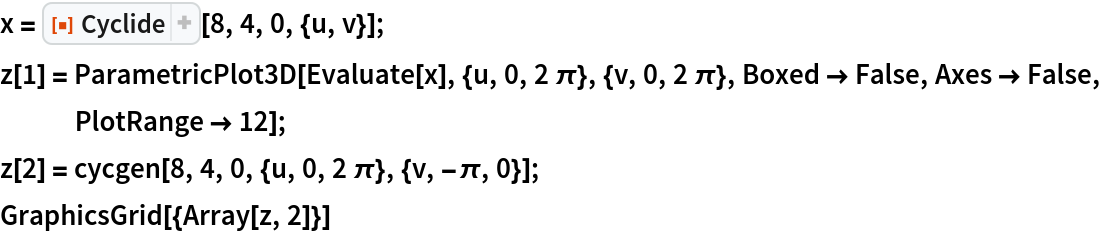Out=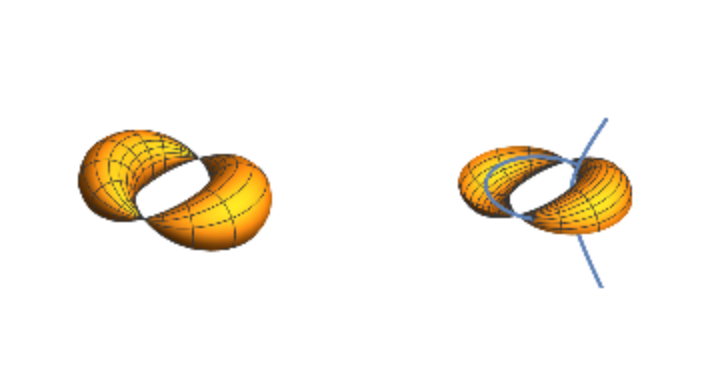The spindle cyclide with its focal curves:

 In:=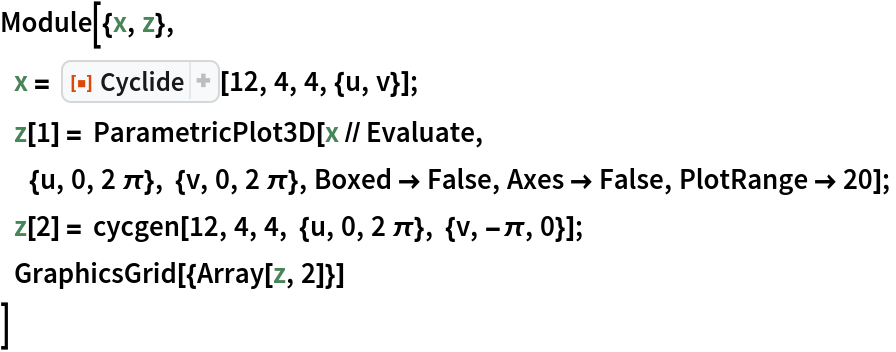Out=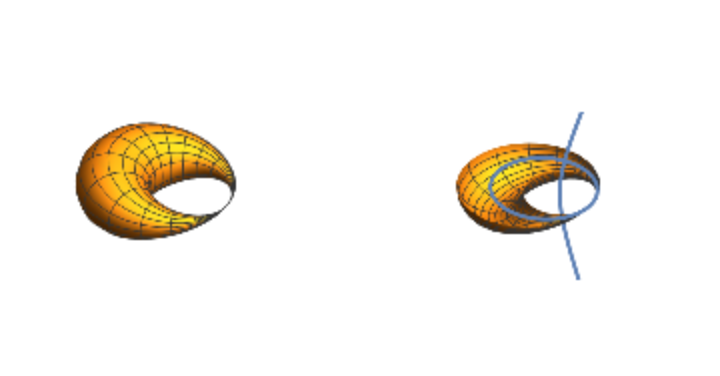A parallel surface of a ring cyclide is another cyclide:

 In:=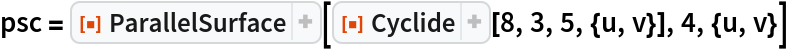Out=In:=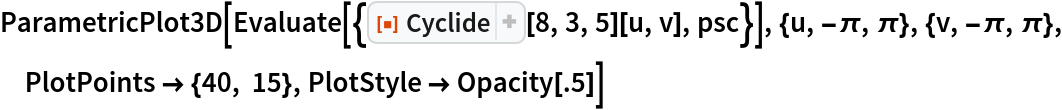Out=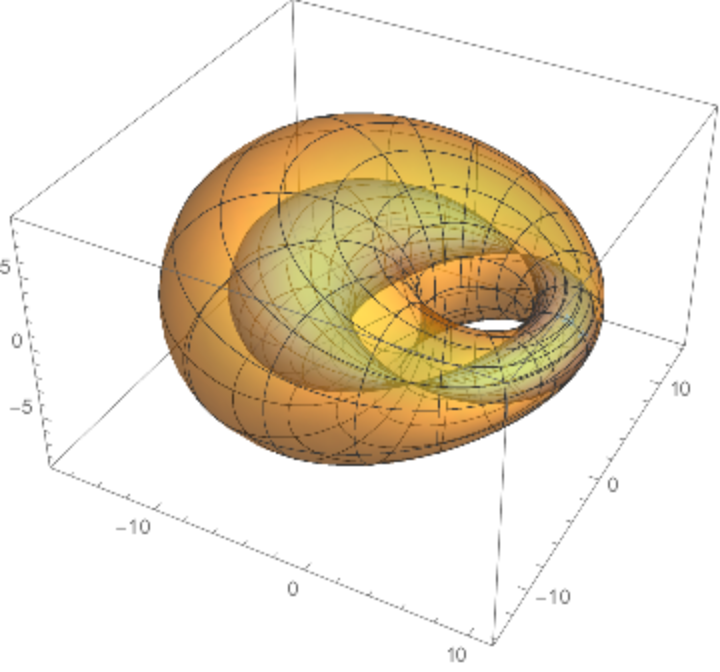The same cyclide with a parallel surface of negative distance:

 In:=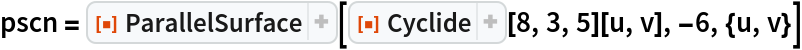Out=In:=Out=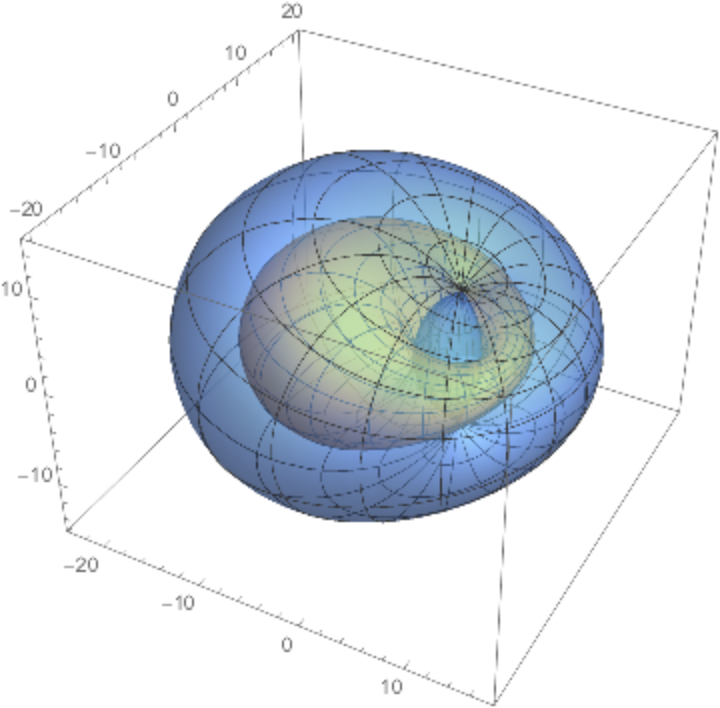Modifying parameter k by n units is equivalent to moving a parallel surface a distance of n units:

 In:=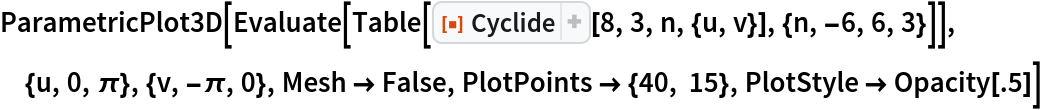Out=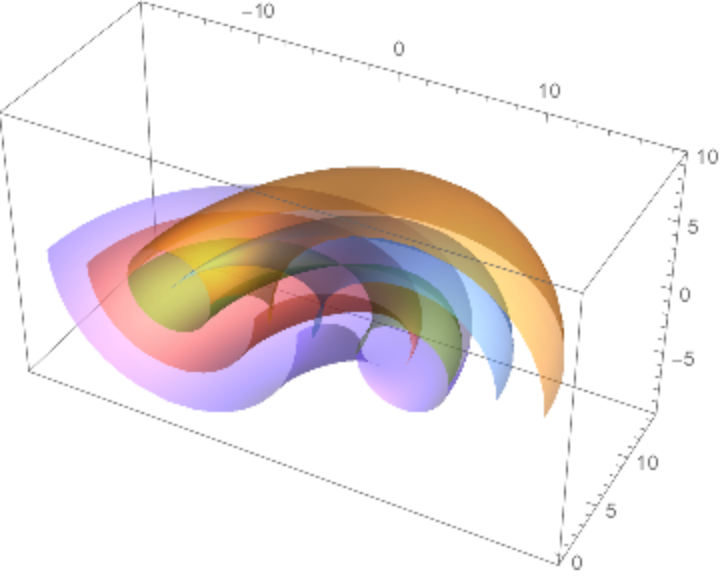Solve the geodesic equations of a ring cyclide:

 In:=Plot the solutions:

 In:=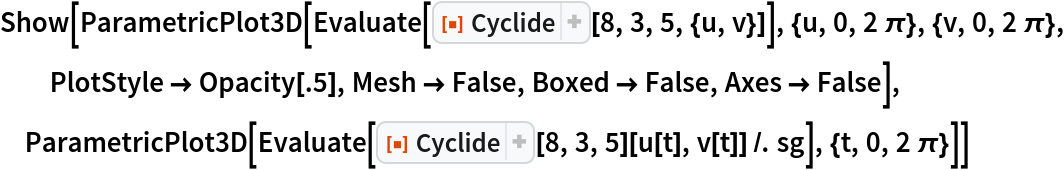Out=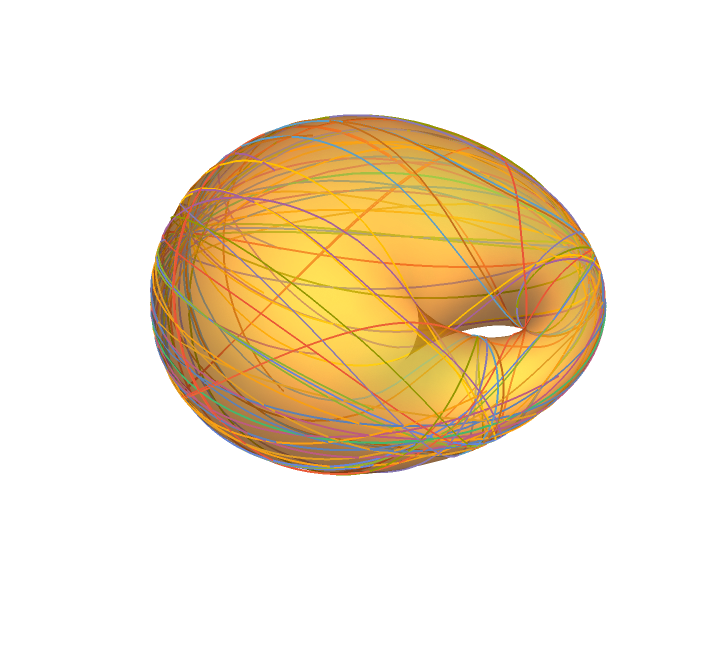Enrique Zeleny

## Version History

• 1.0.0 – 14 July 2020